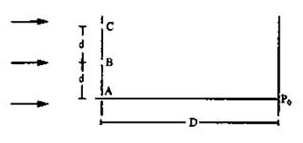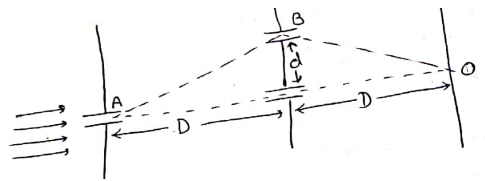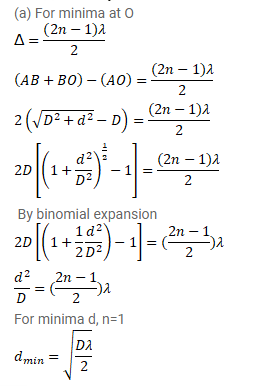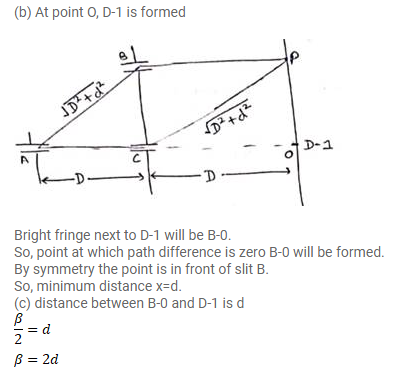# Consider the arrangement shown in figure.Question:

Consider the arrangement shown in figure. The distance $D$ is large compared to the separation $d$ between the slits.

(a) Find the minimum value of $d$ so that there is a dark fringe at $O$.

(b) Suppose $d$ has this value. Find the distance $x$ at which the next bright fringe is formed.

(c) Find the fringe-width.Solution: